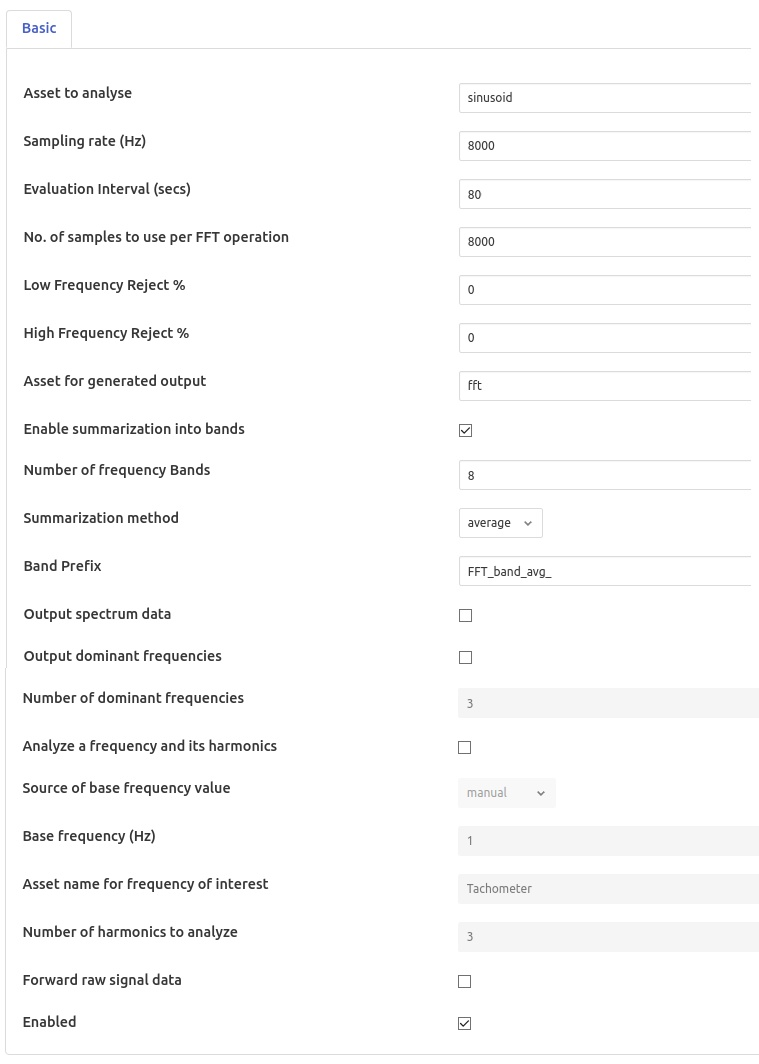# FFT2 Filter¶

The foglamp-filter-fft2 is a filter that applies Fourier Transform to signal data to convert it to frequency domain.

Data is returned in a single reading with a set of datapoints that each represent the average amplitude for a band of frequencies. These bands are created by dividing the frequency space into a number of equal ranges after first applying a low and high frequency filter to discard a percentage of the low and high frequency results.

E.g. if the lowPass filter is set to 15% and the highPass filter is set to 10%, with the number of bands set to 5, the lower 15% of results are discarded and the upper 10% are discarded. The remaining 75% of readings are then divided into 5 equal bands, each of which representing 15% of the original result space. The results within each of the 15% bands are then averaged to produce a result for the frequency band.

It is also possible to get the top ‘k’ dominant frequencies in the input signal. Also one could analyze a base frequency and its harmonics.

FFT2 filter is added in the same way as any other filters.

• Select the fft2 plugin from the list of available plugins.

• Name your FFT filter instance

• Click Next and you will be presented with the configuration page as in the image below. Configure as required.

• Enable the filter and click Done to activate it• Asset to analyse: The asset to apply the FFT filter to

• Sampling rate (Hz): Sampling rate of the input signal (in Hz)

• No. of samples to use per FFT operation: The number of input samples to use. This must be a power of 2.

• Low Frequency Reject %: A percentage of low frequencies to discard, effectively reducing the range of frequencies to examine

• High Frequency Reject %: A percentage of high frequencies to discard, effectively reducing the range of frequencies to examine

• Asset for generated output: The asset name to use for reading containing FFT filter output

• Enable summarization into bands: Whether to summarize the FFT output into bands after applying configured lowPass and highPass

• Number of frequency Bands: The number of frequency bands to split the resultant FFT output into

• Summarization method: The data that should be returned for each band. This should be one of average, sum, peak or rms. Selecting ‘average’ will return the average amplitude within the band, ‘sum’ returns the sum of all amplitudes within the frequency band, ‘peak’ returns the highest amplitude and ‘rms’ returns the root mean square of the amplitudes within the band.

• Band Prefix: Prefix for band datapoints

• Output spectrum data: Output reading would result in the full FFT spectrum data being added to output. Spectrum data however can not be sent to all north destinations as it is not supported natively on all the systems FogLAMP can send data to.

• Output dominant frequencies: Selecting this option results in adding “dominant frequencies” information to output reading.

• Number of dominant frequencies: The number of dominant frequency components to put into output of this filter.

• Analyze a frequency and its harmonics: Selecting this option allows analysis of a base freqeuncy and its ‘k’ harmonics.

• Source of base frequency value: Selects whether the base frequency would be entered manually or it should be picked from an asset value

• Base frequency (Hz): Base frequency value (in Hz)

• Asset name for frequency of interest: If base frequency is to picked from an asset value, that asset’s name

• Number of harmonics to analyze: Number of harmonics of the base frequency to be analyzed

• Forward raw signal data: Selects whether raw signal data should be forwarded towards storage

• Enabled: Whether to enable this filter